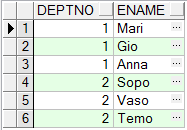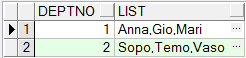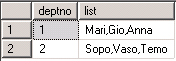# Put Multiple Rows in a Single Row

In my working experience selecting multiple rows into one row was in “high-demand” query. So I decided to write here these queries as in Oracle also in SQL Server 2005:

First of all let’s create table and fill it with desired rows.

————————————————————Oracle—————————————————————–

–Create table

```CREATE TABLE testTable(
deptno number,
ename varchar2(50)
);```

–Fill table with data

```insert into testTable
values(1,'Mari');

insert into testTable
values(1,'Gio');

insert into testTable
values(1,'Anna');

insert into testTable
values(2,'Sopo');

insert into testTable
values(2,'Vaso');

insert into testTable
values(2,'Temo');```

–Let see the data in testTable

```select *
from testTable```

–Output–But  our desired output is the following:–To achieve this,we should write the following query:

```SELECT deptno,
,LTRIM(SYS_CONNECT_BY_PATH(ename,','),',') as list
FROM(SELECT deptno
,ename
,ROW_NUMBER() OVER (PARTITION BY deptno ORDER BY ename) AS seq
FROM  testTable)
WHERE  connect_by_isleaf = 1
CONNECT BY seq = PRIOR seq +1 AND deptno = PRIOR deptno

———————————————————–Sql Server 2005——————————————————-

–Create table

```CREATE TABLE testTable(
deptno numeric(18) ,
ename varchar(50)
);```

–Fill table with data

```insert into testTable
values(1,'Mari');

insert into testTable
values(1,'Gio');

insert into testTable
values(1,'Anna');

insert into testTable
values(2,'Sopo');

insert into testTable
values(2,'Vaso');

insert into testTable
values(2,'Temo');```

–Let see the data in testTable

```select *
from testTable ```

–Output–But  our desired output is the following:–To achieve this,we should write the following query:

```SELECT DISTINCT deptno,
STUFF(value_list, 1, 1, '') AS list
FROM testTable AS A
CROSS APPLY (
SELECT ',' + ename
FROM testTable AS B
WHERE B.deptno =A.deptno
FOR XML PATH('')
) AS t(value_list); ```

–Let’s discuss the following functions:

```*STUFF-It will replace substring started by position 1 with length 1 by '',
which means substring started by position 1 with length 1 will be removed.
Note:  We need to remove first character,because it is extra comma(output was ,Mari...)
* CROSS APPLY-this function will return corresponding rows from right table expression
to left table expression.
* FOR XML PATH-returns a single string in xml format.```About Mariami Kupatadze